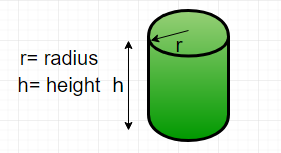# Find the perimeter of a cylinder

Given diameter and height, find the perimeter of a cylinder.

Perimeter is the length of the outline of a two – dimensional shape. A cylinder is a three – dimensional shape. So, technically we cannot find the perimeter of a cylinder but we can find the perimeter of the cross-section of the cylinder. This can be done by creating the projection on its base, thus, creating the projection on its side, then the shape would be reduced to a rectangle.Formula :
Perimeter of cylinder ( P ) =here d is the diameter of the cylinder
h is the height of the cylinder

Examples :

```Input : diameter = 5, height = 10
Output : Perimeter = 30

Input : diameter = 50, height = 150
Output : Perimeter = 400
```

## C++

 `// CPP program to find  ` `// perimeter of cylinder ` `#include ` `using` `namespace` `std; ` ` `  `// Function to calculate perimeter ` `int` `perimeter(``int` `diameter, ``int` `height) ` `{ ` `    ``return` `2 * (diameter + height); ` `} ` ` `  `// Driver function ` `int` `main() ` `{ ` `    ``int` `diameter = 5; ` `    ``int` `height = 10; ` `     `  `    ``cout << ``"Perimeter = "``;  ` `    ``cout<< perimeter(diameter, height); ` `    ``cout<<``" units\n"``; ` `     `  `    ``return` `0; ` `} `

## Java

 `// Java program to find  ` `// perimeter of cylinder ` `import` `java.io.*; ` ` `  `class` `GFG { ` ` `  `    ``// Function to calculate perimeter ` `    ``static` `int` `perimeter(``int` `diameter, ``int` `height) ` `    ``{ ` `        ``return` `2` `* (diameter + height); ` `    ``} ` `     `  `    ``/* Driver program to test above function */` `    ``public` `static` `void` `main(String[] args) ` `    ``{ ` `        ``int` `diameter = ``5``; ` `        ``int` `height = ``10``; ` `        ``System.out.println(``"Perimeter = "` `+ ` `                         ``perimeter(diameter, height)  ` `                                       ``+ ``" units\n"``); ` `    ``} ` `} ` ` `  `// This code is contributed by Gitanjali. `

## Python

 `# Function to calculate  ` `# the perimeter of a cylinder ` `def` `perimeter( diameter, height ) : ` `    ``return` `2` `*` `( diameter ``+` `height )  ` ` `  `# Driver function ` `diameter ``=` `5` `; ` `height ``=` `10` `; ` `print` `(``"Perimeter = "``, ` `            ``perimeter(diameter, height)) `

## C#

 `// C# program to find perimeter of cylinder ` `using` `System; ` ` `  `class` `GFG { ` ` `  `    ``// Function to calculate perimeter ` `    ``static` `int` `perimeter(``int` `diameter, ``int` `height) ` `    ``{ ` `        ``return` `2 * (diameter + height); ` `    ``} ` `     `  `    ``/* Driver program to test above function */` `    ``public` `static` `void` `Main(String[] args) ` `    ``{ ` `        ``int` `diameter = 5; ` `        ``int` `height = 10; ` `        ``Console.Write(``"Perimeter = "` `+ ` `                       ``perimeter(diameter, height)  ` `                                    ``+ ``" units\n"``); ` `    ``} ` `} ` ` `  `// This code is contributed by parashar... `

## PHP

 ` `

Output :

```Perimeter = 30 units
```

Don’t stop now and take your learning to the next level. Learn all the important concepts of Data Structures and Algorithms with the help of the most trusted course: DSA Self Paced. Become industry ready at a student-friendly price.

My Personal Notes arrow_drop_upCheck out this Author's contributed articles.

If you like GeeksforGeeks and would like to contribute, you can also write an article using contribute.geeksforgeeks.org or mail your article to contribute@geeksforgeeks.org. See your article appearing on the GeeksforGeeks main page and help other Geeks.

Please Improve this article if you find anything incorrect by clicking on the "Improve Article" button below.

Improved By : parashar, vt_m